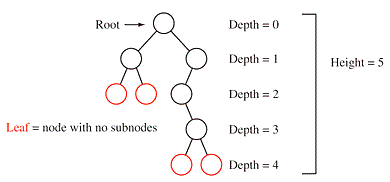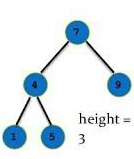# Find Height (Maximum Depth) of a Binary Search Tree (C++ program)

Learn: How to find the height or maximum depth of a binary search tree? This article includes definition, algorithm and implementation in C++ program.
Submitted by Abhishek Jain, on July 30, 2017

The Height (or depth) of a tree is defined to be the maximum level of any node in the tree. Some authors define depth of a node to be the length of the longest path from the root node to that node, which yields the relation:

Depth of the tree = Height of the tree - 1Reference: http://codersmaze.com/data-structure-explanations/trees-in-data-structure/

Algorithm:

```FindHeight( Node root)
If root=NULL
return 0
Else
int l=FindHeight (root->left)
int r=FindHeight(root->right)
Return max(l,r)+1
[End If]
[End]
```Consider the program:

```#include<iostream>
using namespace std;

struct node
{
int data;
node* left;
node* right;
};

struct node* getNode(int data)
{
node* newNode=new node();
newNode->data=data;
newNode->left=NULL;
newNode->right=NULL;
return newNode;
}

void inorder(struct node* root)
{
if (root != NULL)
{
inorder(root->left);
cout<<root->data<<" ";
inorder(root->right);
}
}

struct node* Insert(struct node* root, int data)
{
if (root == NULL)
return getNode(data);

if (data < root->data)
root->left  = Insert(root->left, data);
else if (data > root->data)
root->right = Insert(root->right, data);

return root;
}

int FindHeight(node* root)
{
if(root==NULL)
return 0;

else
{
int lb=FindHeight(root->left);
int rb=FindHeight(root->right);
return max(lb,rb)+1;
}
}
int main()
{
node* root=NULL;
root=Insert(root,7);
Insert(root,9);
Insert(root,4);
Insert(root,1);
Insert(root,5);

cout<<"Inorder: ";
inorder(root);
cout<<endl;

cout<<"Height of the tree is "<<FindHeight(root)<<endl;
cout<<"Max. Depth of the tree is "<<FindHeight(root)-1;

return 0;
}
```

Output

```Inorder: 14579
Height of the tree is 3
Max. Depth of the tree is 2
```

Top MCQs

Languages: » C » C++ » C++ STL » Java » Data Structure » C#.Net » Android » Kotlin » SQL
Web Technologies: » PHP » Python » JavaScript » CSS » Ajax » Node.js » Web programming/HTML
Solved programs: » C » C++ » DS » Java » C#
Aptitude que. & ans.: » C » C++ » Java » DBMS
Interview que. & ans.: » C » Embedded C » Java » SEO » HR
CS Subjects: » CS Basics » O.S. » Networks » DBMS » Embedded Systems » Cloud Computing
» Machine learning » CS Organizations » Linux » DOS
More: » Articles » Puzzles » News/Updates